# Scouts

Three scouts went on a three day trip. The second day went 4 km more than the first day. The third day went two times less than the first day. They went along 54 km. How many kilometers went every day together?

Correct result:

a =  20 km
b =  24 km
c =  10 km

#### Solution:

b = 4+a
c = a/2
a+b+c = 54

a-b = -4
a-2c = 0
a+b+c = 54

a = 20
b = 24
c = 10

Our linear equations calculator calculates it.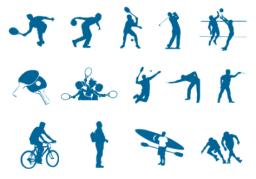We would be pleased if you find an error in the word problem, spelling mistakes, or inaccuracies and send it to us. Thank you!Tips to related online calculators
Do you have a linear equation or system of equations and looking for its solution? Or do you have quadratic equation?
Do you want to convert time units like minutes to seconds?

## Next similar math problems:

• Scouts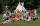The boys from scout group traveled 5 days distance 115 km. Every day walked 1.5 km less than the previous day. How many kilometers scouts walked in the first day?
• Three-day tripThe cyclist on three-day trip travel 30% of the total route on the first day, 3/5 of the rest on the second day and 35 km on the third day. How many kilometers did travel cyclists each day and how many?
• Cyclist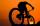Cyclist rode two-fifths of the way the first day. The second day drove 5 km less while traveled three-eighths of route. How many kilometers does he have to finish target?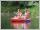Students of canoeists trip traveled in three days 102 km. Second day traveled 15% more than first day and at the third day 9 km over the second day. How many kilometers traveled each day?
• Journey to school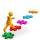On the way to school I went steady step. In the first half of the journey, I counted every second step, in the second half every third. How many steps I do when I went to school if I counted double step are 250 more than three steps?
• Hiker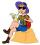Hiker went half of trip the first day, the third of trip the second day and remaines 15 km. How long trip he planned?
• Three daysDuring the three days sold in stationery 1490 workbooks. The first day sold about workbooks more than third day. The second day 190 workbooks sold less than third day. How many workbooks sold during each day?
• The hikerThe hiker will travel 40% of the route on the first day 1and/3 of the rest od second day. Last day 30 km. What was the length of the 3-day trip? How many kilometers did he walk each day?
• PotatoesFor three days the store sold 1400 kg of potatoes. The first day they sold 100 kilograms of potatoes less than the second day, the third-day three-fifths of what they sold the first day. How many kgs of potatoes sold every day?
• Bags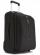The first bag has a weight of 18 kg, the second bag is a one-third lighter than the first and the third bag has three times less the weight of all three bags together. Determine their weights.
• Scratches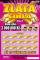The ticket seller sold 1,280 scratch cards in three days. On the second day, he sold 90 tickets less than on the first day, on the third day he sold 1.5 times more tickets than on the second day. How many tickets did he sell on the third day?
• Three workersThree workers were rewarded CZK 9200 and the money divided by the work they have done. First worker to get twice than the second, the second three times more than the third. How much money each worker received?
• TrucksThree lorries droved bricks. One drove n bricks at once, second m less bricks than the first and third 300 bricks more the first lorry. The first lorry went 4 times a day the largest went 3 times a day and the smallest 5 times a day. How many bricks broug
• Stamps and albumsMichail has in three albums stored 170 stamps. In the first album, there are 14 more than in the second and in the second is by 1/5 less than in the third. How many stamps are in the first album?
• Tourists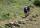From the cottage started first group of tourists at 10:00 AM at speed 4 km/h. The second started after them 47 minutes later at speed 6 km/h. How long and how many kilometers from the cottage will catch the first group?
• Three workers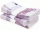The three workers received € 2,850 together for the work done. They divided them according to the time worked so that the first received 20% less than the second and the third € 50 more than the second. How much EUR did each worker receive?
• Three friends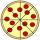Divide 570 euros to three friends so that first will get 50 euros less than the second and third twice more than the first. How many euros will get everyone?